Courses
Courses for Kids
Free study material
Free LIVE classes
More

# How to Solve 2 Digit Multiplication ProblemsLIVE
Join Vedantu’s FREE Mastercalss

## Understanding Double Digit Multiplication with Solved Examples

Multiplication is one of the easiest mathematical operations and it is all about combining numbers or other quantities under specific rules to obtain their product. It's a process of repeated addition of a number with respect to the other number. For example, 6X5 means we add 6, 5 times; 6+6+6+6+6=30. This addition process is very complicated and therefore these multiplication methods come in handy.

Before we start, we should at least know the basics of multiplication which are

1. Multiplicand (the first number) X Multiplier (the second number) = Product

2. Any number multiplied by 0 makes the product equal to 0. e.g.- 5x0=0

3. Any number multiplied by 1 equals the same number. e.g.- 5x1=5

## Three Simple Methods of Double Digit Multiplication

There are 3 main methods of 2-digit by 2-digit multiplication, which are discussed below.

2. Box/window method

3. Lattice method

## 1. Using the Traditional Method

25X42=?

Step 1: Set the problem up. So, we will have to align them according to their place value.

Step 2: Multiplying the number in the one's place and tens place of the multiplier with the entire multiplicand separately.

Note: We need to put 0 before we do the second digit of the multiplier.

Step 3: Add both the values which we got from multiplying separately.

carried = 5Step 1 of Multiplication

5X2 = 10, 1 will be carried forward to the tens place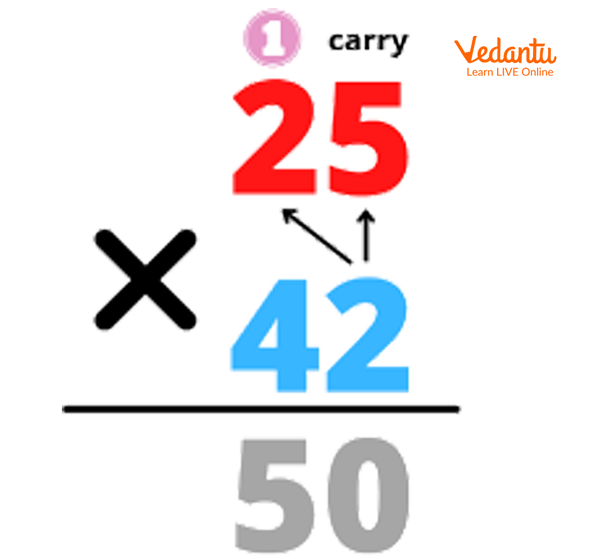Step 2 of Multiplication

2X2 = 4 and +1 that we

4X5 = 20, 2 carried forward

4X2 = 8 and +2 (carry)= 10. We drop down the 1 as well because there is no further number available to carry.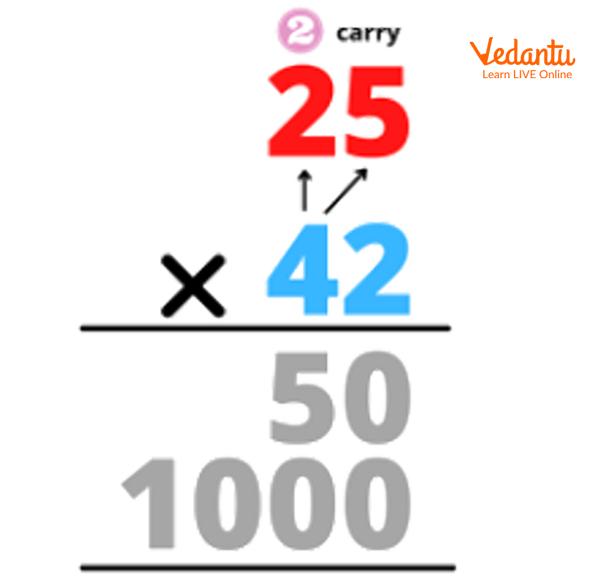Step 3 of Multiplication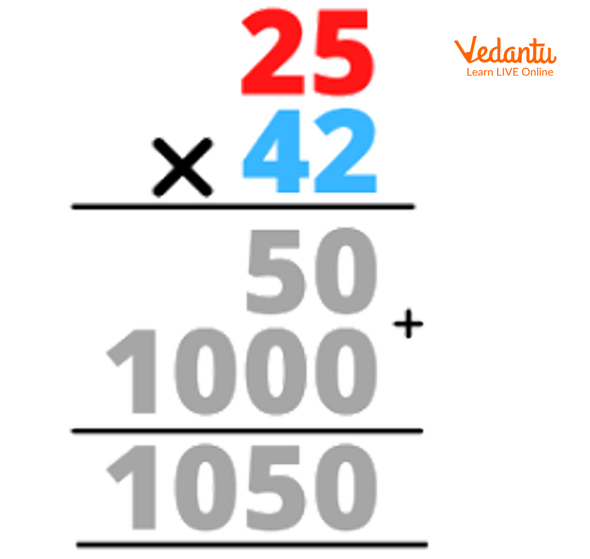Step 4 of Multiplication

50+1000 we get 1050 which is our final product.

### 2.Box/Window Method

32X34=?

Step 1: Make a box table or a grid of 2X2 as we are doing a 2-digit multiplication equation.

Step 2: Breaking these factors up into their expanded forms. So, 32 becomes 30 and 2; 34 becomes 30 and 4.

Note: Label the expanded form of the multiplicand on the top and the expanded form of the multiplier on the left-hand side of the grid.

Step 3: We multiply the numbers that meet in each space on the box. Follow the image above.

Step 4: Add all those small products that we got in order to obtain the final product.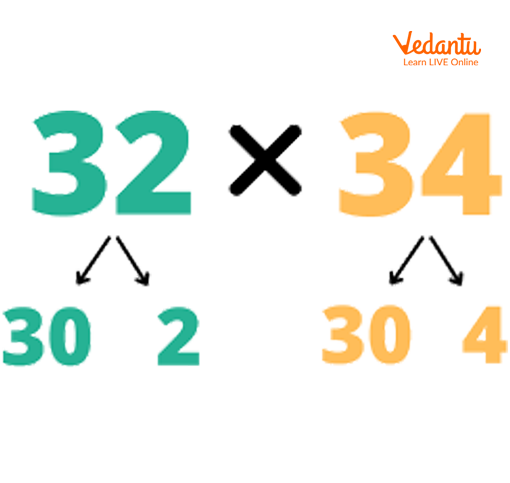Expanded forms of Factors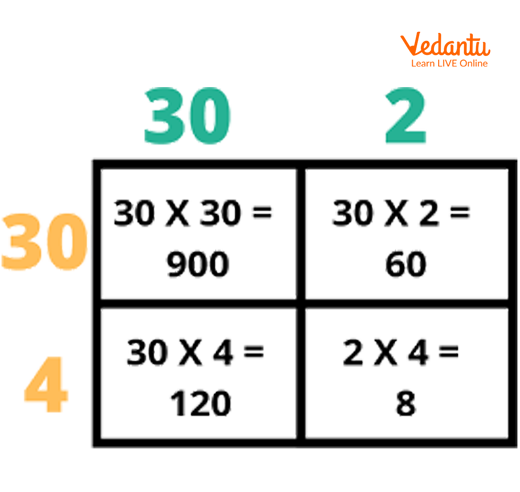Step 2 of the box Method

900+60+120+8=1088 -> Final Product

## 3. Lattice Method

25X42=?

Step 1: Make a grid that matches according to the number of digits required. In this case, we require a 2X2 grid as we are doing 2 digits multiplication.

Note: There is no need to expand the factors so we directly label the multiplicand on top of the grid and the multiplier on the right-hand side of the grid.

Step 2: Multiply the numbers that meet in each space and write the tens place value of the product on the top of the box and the ones place value of the same on the bottom of the box.

Step 3: Adding the numbers which are in the same lane.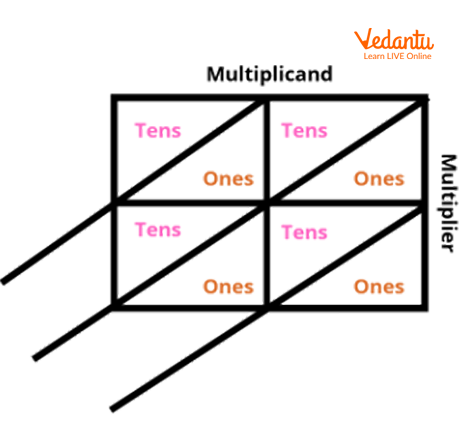Step 1 of the Lattice Method

5X4=20

2X4=08 (we put 0 in the tens place)

5X2=10

2X2=04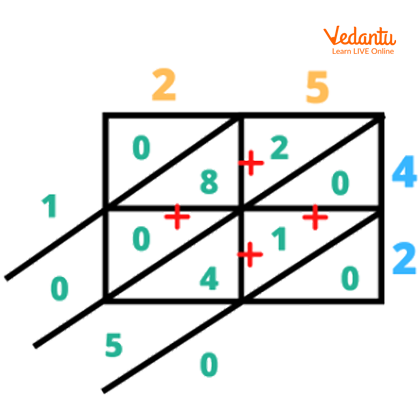Steps of Lattice Method

We put 0 as it is because there is no other number to add it with.

4+1+0=5

0+8+2=10 (1 has been directly put into the thousands place)

So, our final product is 1050.

## Solved Examples

Below are some of the 2-digit by 2-digit multiplication problems:

Q1. 98X66=?

Solution: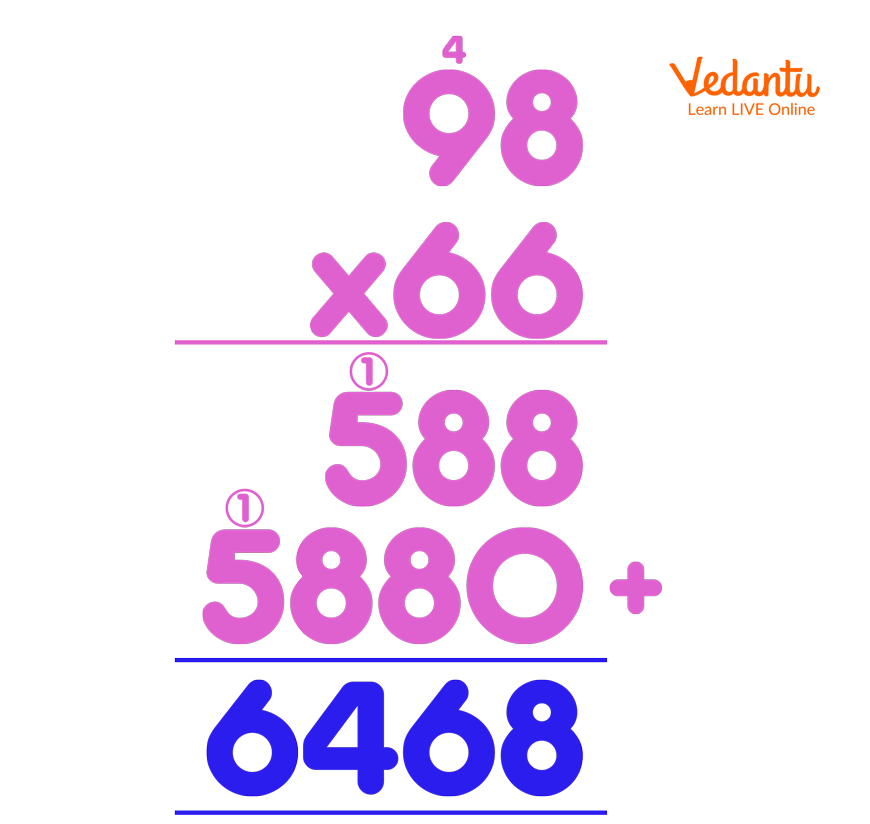Solution of Q 1

Q2. 75X39=?

Solution: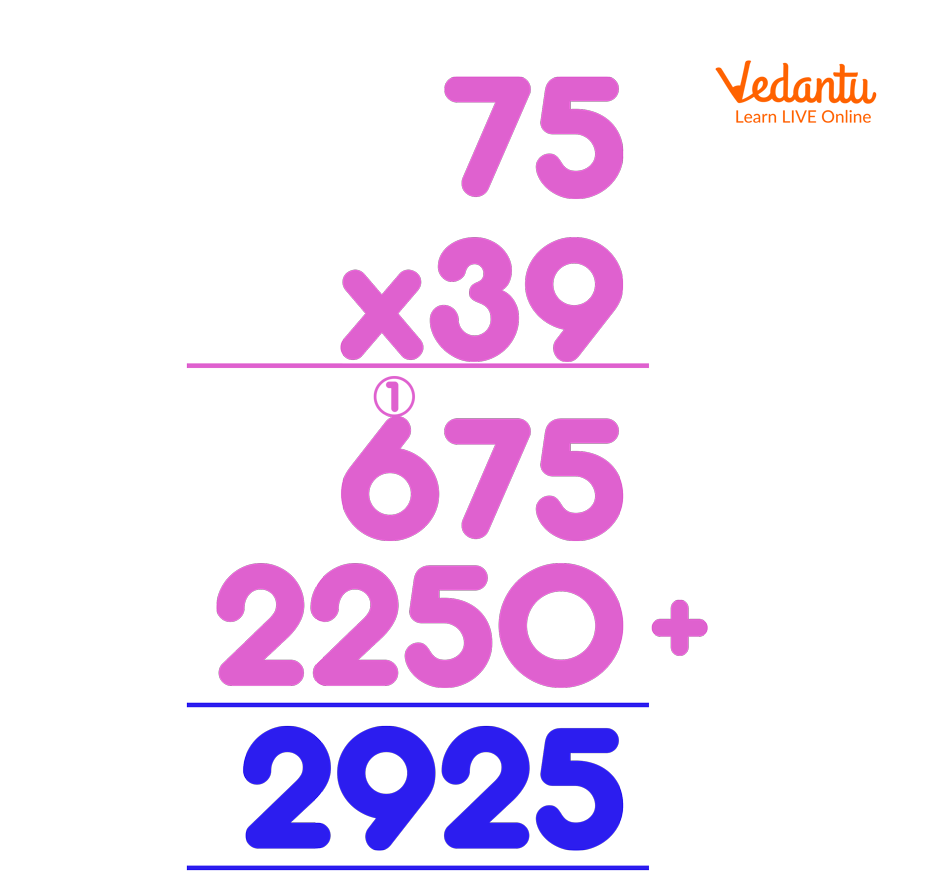Solution of Q 2

Q1. 62X70=

Ans: 4340

Q2. 49X10=

Ans: 490

Q3. 27X19=

Ans: 513

## Summary

In this article, we were able to understand 3 main methods which can be used to multiply numbers which are of 2 digits. If we see, the traditional method is handier and is very easy to multiply numbers that are more than 2 digits as well. We also understood the steps involved in all the 3 methods with the help of images that had the procedures illustrated. You can visit our website to download and practise some multiplication two-digit numbers worksheets for better understanding.

Last updated date: 21st Sep 2023
Total views: 110.1k
Views today: 3.10k

## FAQs on How to Solve 2 Digit Multiplication Problems

1. What is a multiplication wheel?

Multiplication wheel is the easiest way to learn times tables for beginners who are very new to multiplication. It is a method where the number (multiplicand) written at the centre of the wheel is multiplied by the numbers which are surrounding it (multiplier) and the product is written in the blanks.  This method speeds up the ability to memorise single-digit multiplication. Teachers often make it interesting by using artistic and crafty methods to explain the multiplication wheel for better understanding and learning.

2. What is the commutative law of multiplication?

According to the commutative law of multiplication and addition, the product of two integers will not change regardless of their positionings. The product of two numbers will stay the same even if their positions are interchanged. For example, 6X5=5X6=>30 (in the case of multiplication) and 6+5=5+6=>11 (in the case of addition).  It is called commutative because the numbers can travel back and forth or be swapped and still will have the same answer.

3. What is the formula for multiplying a decimal by a whole number?

Performing regular multiplication without considering the decimal point at the beginning is similar to multiplying a decimal by a whole number. After analysing the numbers, the decimal point is placed according to their decimal places. Therefore, all multiplicands and multipliers in the product must be placed in a way that makes the decimal point equal to the sum of the decimal places in the product.